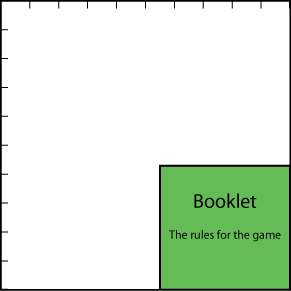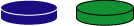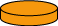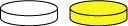#### You may also like### Chocolate

There are three tables in a room with blocks of chocolate on each. Where would be the best place for each child in the class to sit if they came in one at a time?### Four Triangles Puzzle

Cut four triangles from a square as shown in the picture. How many different shapes can you make by fitting the four triangles back together?### Cut it Out

Can you dissect an equilateral triangle into 6 smaller ones? What number of smaller equilateral triangles is it NOT possible to dissect a larger equilateral triangle into?

# Fractions in a Box

## Fractions in a Box

We have a game which has a number of discs in seven different colours. These are kept in a flat square box with a square hole for each disc. There are $10$ holes in each row and $10$ in each column. So, there would be $100$ discs altogether, except that there is a square booklet which is kept in a corner of the box in place of some of the holes.

We haven't drawn a grid to show all the holes because that would give the answer away!There is a different number of discs of each of the seven colours.

Half ($\frac{1}{2}$ ) of the discs are red, $\frac{1}{4}$ are black and $\frac{1}{12}$ are blue.One complete row (of $10$ holes) of the box is filled with all the blue and green discs.One of the shortened rows (that is where the booklet is) is exactly filled with all the orange discs.Two of the shortened rows are filled with some of the red discs and the rest of the red discs exactly fill a number of complete rows (of $10$) in the box.

There is just one white disc and all the rest are yellow.How many discs are there altogether?
What fraction of them are orange?
What fraction are green? Yellow? White?

### Why do this problem?

This problem gives practice in calculating with fractions in a challenging setting. It also requires the use of factors and multiples. While doing the problem learners will need to express a smaller whole number as a fraction of a larger one and find equivalent fractions. This activity will require some estimating and trial and improvement, combined with working systematically.

### Possible approach

You could start by showing the picture in the problem and explaining the task orally to the group. Give them a chance to think on their own for a minute then ask them to talk to a partner about how they might start the problem. Sharing some ideas will help you ascertain whether learners understand the task and it will give you the chance to talk through any misconceptions they may have. It may be helpful to collate some known facts or suggestions on the board. At this stage, welcome all ideas for how to begin as long as they are backed up by logical reasons. It is likely that many will involve testing a size for the booklet, but different children may have different starting points for the size. Others might want to test total numbers of counters.

After that encourage them to work in pairs on the problem from a printed sheet (this sheet is photocopiable) so that they are able to talk through their ideas with a partner. Make sure that learners have access to any resources that they require, such as squared paper, coloured pens/pencils, mini-whiteboards, plain paper, counters ... Warn them that you would like them to be able to explain how they approached the problem at the end of the lesson. You could ask each pair or group to produce a poster of their working.

In the plenary, you could invite the class to look at each other's posters and ask each other questions about the methods used.

### Key questions

What shape is the booklet? How are you going to work out its size?
How many holes could the booklet could take up?
What do you know about the factors of the total number of discs?

### Possible extension

Learners could find out possible fractions for the differently coloured discs if the square booklet was larger, say $6 \times 6$.

### Possible support

Some children my find it helpful for you to structure the approach a little. You could suggest making a list of the possible sizes for the square booklet then working out the number of remaining small square holes for the coloured discs.